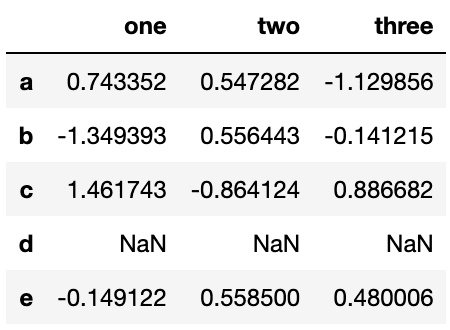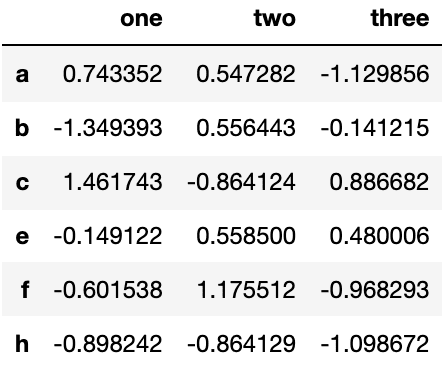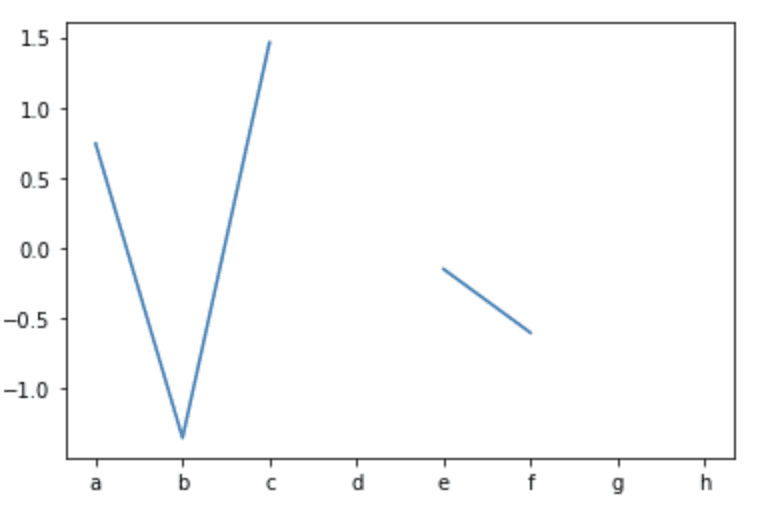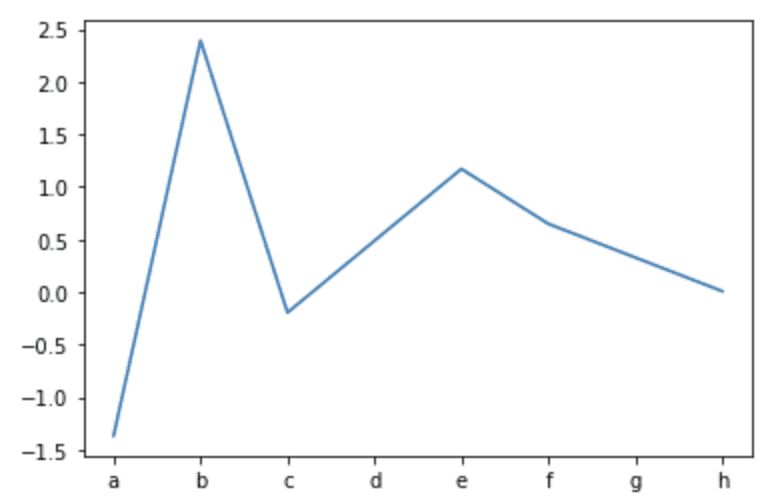# Getting Started With Pandas: Lesson 4

## Introduction

We begin with the fourth and final article of our saga of training with Pandas. In this article, we are going to make a summary of the different functions that are used in Pandas to perform missing data treatment. Dealing with missing data is key and a standard challenge of day-by-day data science work, and it has a direct impact on algorithmic performance.

## Missing Data

Before we start, we are going to visualize the example dataset that we are going to follow to explain the functions. It is a dataset created by us that includes several cases of use to be able to clearly deal with all the examples that we will call `uncompleted_data`.It is important to clarify what the missing data is and how it is identified. We have different values ​​for this:

+ Nan in numeric arrays

+ None or NaN in object arrays

+ Nat in datetime objects

### Isna

We start with the isna() function. This function takes a scalar or array-like object and indicates whether values ​​are missing. For scalar input, it returns a scalar boolean. For array input, returns an array of booleans indicating whether each corresponding element is missing.

In this case, we are going to detect missing values ​​in column `one` using the `isna()` function:

``````uncompleted_data['one'].isna()

a    False
b    False
c    False
d     True
e    False
f    False
g     True
h    False
Name: one, dtype: bool``````

### Notna

We continue with the notna() function. This function takes a scalar or array-like object and indicates whether values ​​are valid (not missing). For scalar input, returns a scalar boolean. For array input, returns an array of booleans indicating whether each corresponding element is valid. In a nutshell, this is the inverse operation of isna().

For example, we are going to detect missing values ​​in column `one` using the `notna()` function:

``````uncompleted_data['one'].notna()

a     True
b     True
c     True
d    False
e     True
f     True
g    False
h     True
Name: one, dtype: bool``````

Pay attention to the fact that isna() and notna() are totally opposite depending on the functionality you want to achieve, and the common thing they have is that both return a boolean.

### Fillna

This function is one of the most used in data cleaning because it is useful for the search and replacement of the values ​​considered missing values.

We are going to show two very common examples of replacements. The first is to replace for a specific value passed as an argument and the second is for a calculated value from the dataset, such as mean. We could also fill it with other values, such as the mode.

+ Replace missing values ​​by 0:

``````uncompleted_data['one'].fillna(0)

a    0.743352
b   -1.349393
c    1.461743
d    0.000000
e   -0.149122
f   -0.601538
g    0.000000
h   -0.898242
Name: one, dtype: float64``````

+ Replace missing values ​​by mean:

``````uncompleted_data['one'].fillna(uncompleted_data['one'].mean())

a    0.743352
b   -1.349393
c    1.461743
d   -0.132200
e   -0.149122
f   -0.601538
g   -0.132200
h   -0.898242
Name: one, dtype: float64``````

### Dropna

This function is used to remove the rows or columns that contain missing values. This function will drop all rows that contain any missing values ​​in any of the columns.

+ Delete rows containing missing values:

``uncompleted_data.dropna(axis=0)``+ Delete columns containing missing values:

``uncompleted_data.dropna(axis=1)``### Interpolate

This function is used to replace missing values ​​using an interpolation method. Interpolation is a type of estimation, a method of constructing (finding) new data points based on the range of a discrete set of known data points.

In this example, we are going to replace the missing values ​​of column `one` using the method interpolation `linear` by default.Fig 1. Print of data Before and after Interpolation. The interpolation function generates data following a pattern inside the range of known data.

+ After

``uncompleted_data['one'].interpolate()``

### Replace

This function, as its name suggests, is used to replace one value with another, but in this case, we are going to explain one of its most useful uses. It is based on the use of this function in combination with regular expressions.

In this example, we are going to replace by np.nan all the values ​​that are within the range [0,1]:

``````to_replace.replace(r"^0.[0-9]*", np.nan, regex=True)

a                    NaN
b     -1.349393388281627
c     1.4617427030378465
d                    nan
e    -0.1491215416722299
f    -0.6015382564614734
g                    nan
h    -0.8982420093440403
Name: one, dtype: object``````